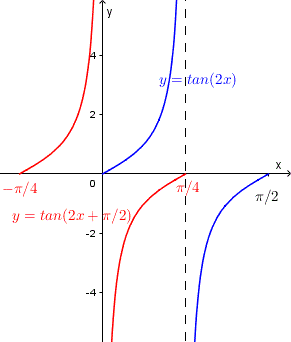# Graph tangent and cotangent

The sketching and graphing of the tangent and cotangent functions of the form

y = a tan [ k ( x - d) ]    and    y = a cot [ k ( x - d) ]
are discussed with detailed examples.

## Graphing Parameters of y = tan(x) and y = cot(x)

range: (-∞ , +∞)
Period = π
Horizontal Shift (translation) = d , to the left if (- d) is positive and to the right if (- d) is negative.
Vertical asymptotes of y = tan(x) at x = π/2 + kπ , k = 0 , ~+mn~1, ~+mn~2, ...
Vertical asymptotes of y = cot(x) at x = kπ , k = 0 , ~+mn~1, ~+mn~2, ...
We need to know how to sketch basic tangent and cotangent functions using the identities y = tan(x) = sin(x) / cos(x) and y = cot(x) = sin(x) / cos(x) to understand certain properties.

## 1) y = tan(x) = sin(x) / cos(x)

All zeros of the sin(x) are also zeros of tan(x) and all zeros of cos(x) (which is in the denominator) are vertical asymptotes of the tan(x) as shown below.## 2) y = cot(x) = cos(x) / sin(x)

All zeros of the cos(x) are also zeros of cot(x) and all zeros of sin(x) (which is in the denominator) are vertical asymptotes of the cot(x) as shown below.## Sketching tangent and cotangent Functions: Examples with Detailed Solutions

### Example 1

Sketch the graph of y = tan(2x + π/2) over one period.
Solution
Graphing Parameters
range: (-∞ , +∞)
Period = π/|k| = π/2
Vertical asymptotes are found by solving for x the equation: 2x + π/2 = π/2 + kπ which gives x = kπ/2 , k = 0 , ~+mn~1, ~+mn~2, ...
Horizontal Shift: Because of the term π/2, the graph is shifted horizontally. We first rewrite the given function as: y = tan [ 2( x + π/4)] and we can now write the shift as being equal to π/4 to the left.
We start by skeching tan(2 x) over one period from 0 to π/2 (blue graph below).
We then sketch y = tan [ 2( x + π/4)] translating the previous graph π/4 to the left (red graph below) so that the sketched period starts at - π/4 and ends at - π/4 + π/2 = π/4 which is an interval over one period equal to π/2.### Example 2

Sketch the graph of y = cot(4x - π/4) over one period.
Solution
Graphing Parameters
range: (-∞ , +∞)
Period = π/|k| = π/4
Vertical asymptotes are found by solving for x the equation: 4x - π/4 = kπ which gives x = (kπ + π/4) / 4 , k = 0 , ~+mn~1, ~+mn~2, ...
Horizontal Shift: Because of the term - π/4, the graph is shifted horizontally. We first rewrite the given function as: y = cot [ 4( x - π/16)] and we can now write the shift as being equal to π/16 to the right.
We start by skeching cot(4 x) over one period from 0 to π/4 (blue graph).
We then sketch y = cot [ 4( x - π/16)] translating the previous graph π/16 to the right (red graph below) so that the sketched period starts at π/16 and ends at π/16 + π/4 = 5π/16 which is one period.